# [Linear Algebra] Basis, Linear Inependence

## Homework Statement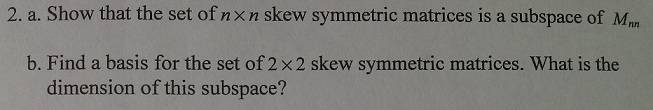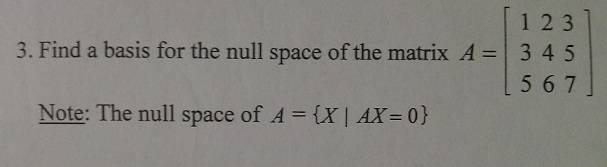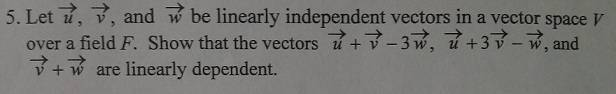## The Attempt at a Solution

2) No clue.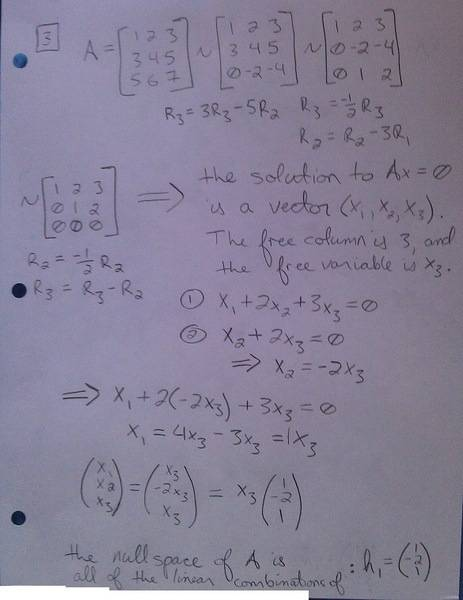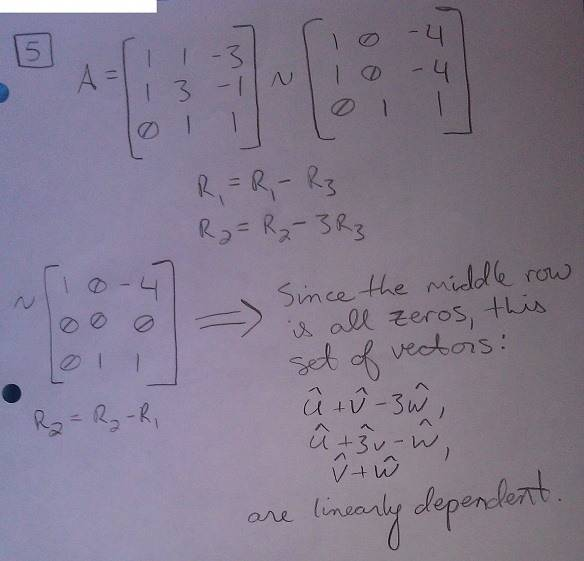2a) I found this, and see that it is taking the definition of a symmetric matrix, and adding it to a skew-symmetric matrix to show that it forms a subspace by definition.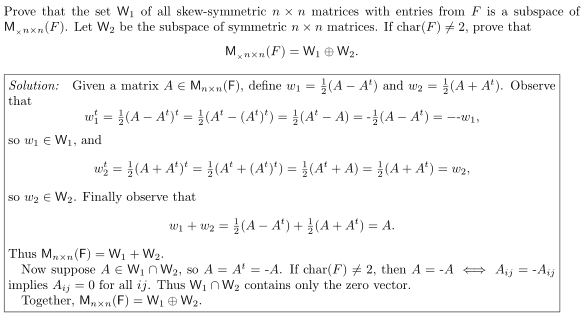I am not sure if this is what I have to do. . .

2b) I am not familiar with basis other than S = {v1, v2, ..., vr} being independent and S spanning V.

3) Apparently the question asks for the BASIS of the nullspace, and not the nullspace which I have found. . .

5) My professor told me that I need to be more clear on showing where those numbers and sets of vectors came from in my solution, but I am out of ideas. He mentioned that I would want to do something like: c1v1 + c2v2 + c3v3 = 0

Deveno
for (2), do you know how you check that a subset S of a vector space V is a subspace?

there are 2 closure conditions, and a third condition...any guess as to what these are?

as for finding a basis, can you think of linear combinations of a basis for Mnxn that might be symmetric matrices?

do you know of any bases for Mnxn (hint: think of a matrix as being n n-vectors laid "end-to-end"...what is an obvious basis for Rn2?)

(3) you're close. suppose that every element of a subspace is a multiple of some vector v. prove that {v} is a basis for that subspace.

(5) if you keep careful track of which row-operations you performed, these will give you a way to construct a linear combination of the 3 vectors you started out with. what exactly did you do to row 2, that made it 0? express that as an equation involving u,v and w.

for (2), do you know how you check that a subset S of a vector space V is a subspace?

there are 2 closure conditions, and a third condition...any guess as to what these are?

It needs to be shown that they hold under addition, multiplication, and that the zero vector is contained in the set. . .

Deveno
yes. so...how do you show that the sum of two skew-symmetric matrics is skew-symmetric?

yes. so...how do you show that the sum of two skew-symmetric matrics is skew-symmetric?

it's a theorem or definition in the book. . . you would just make up two arbitrary ones and show that it holds, right?

as for finding a basis, can you think of linear combinations of a basis for Mnxn that might be symmetric matrices?

do you know of any bases for Mnxn (hint: think of a matrix as being n n-vectors laid "end-to-end"...what is an obvious basis for Rn2?)

(3) you're close. suppose that every element of a subspace is a multiple of some vector v. prove that {v} is a basis for that subspace.

I know what you are saying, but I'm having trouble figuring out how I would exactly do that. I know what you are saying to do, and how/why that works. . .

(5) if you keep careful track of which row-operations you performed, these will give you a way to construct a linear combination of the 3 vectors you started out with. what exactly did you do to row 2, that made it 0? express that as an equation involving u,v and w.

I have my 3 row operations listed, but I'm not sure how I would write that up. . . it seems like it would make more sense to re-do the reduction with column operations, so that everything would be in terms of u, v, w alone. . .

Deveno
it's a theorem or definition in the book. . . you would just make up two arbitrary ones and show that it holds, right?

the key is you have to use the definition of skew-symmetric somehow. i do not know the definition of skew-symmetric you are using, the easiest one to use in my opinion is:

AT = -A, or A + AT = 0

if this is true for A and B, what can you say about (A+B)T?

to show a set is a basis, you need to show 2 things:

a) the set is linearly independent
b) the set spans the subspace.

if we have a one-element set {v}, showing spanning should be easy, there's only one kind of linear combination: av (there's no other basis elements to add). for a one-element set, linear independence means showing av = 0 implies a = 0.

I know what you are saying, but I'm having trouble figuring out how I would exactly do that. I know what you are saying to do, and how/why that works. . .

I have my 3 row operations listed, but I'm not sure how I would write that up. . . it seems like it would make more sense to re-do the reduction with column operations, so that everything would be in terms of u, v, w alone. . .

yes, you could use column operations. both methods should give you an equation of the form:

(some stuff)u + (other stuff)v + (still more stuff)w = ?

remember, in any linear combination, you can always "collect like terms".

2a) I am not sure if this is the proper way to show addition and multiplication... also, I am not sure how to show that the zero vector is included in this subset... is the 2x2 zero matrix skew-symmetric... I'll check to see if that is the case now.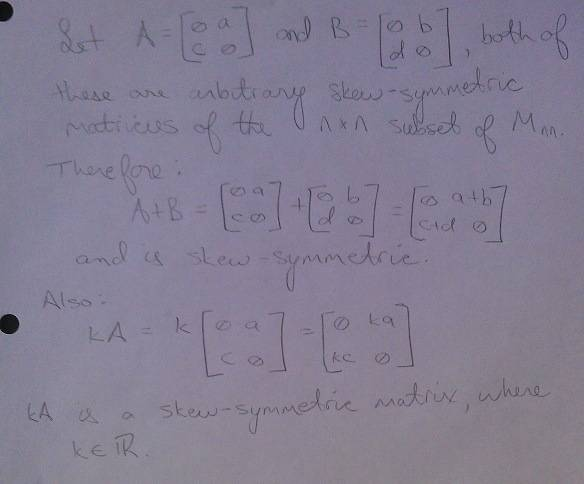Deveno
i thought i'd add a little bit here, about what a basis IS, and why they are so important.

think of a typical vector in R3. well, generically, it's just a point in 3-space, and there are soooo many. so how do we get a handle on telling all these points apart?

one way, is to assign a point a set of coordinates: v = (x,y,z). now we've cut the infinity of points down to just something requiring 3 numbers: x, y and z (the 3 in R3 might have been a dead give-away, huh?).

let's relate this to a basis: we "split the coordinates" like this:

(x,y,z) = x(1,0,0) + y(0,1,0) + z(0,0,1).

so our "x's" are just x times the basis vector (1,0,0), and similarly with the y's and z's.

the set {(1,0,0),(0,1,0),(0,0,1)} has certain properties, which make it useful:

it is linearly independent (you can't "cancel y's" by adding in "x's" and "z's").

it spans all of R3: given these 3 vectors, we can label any point in R3, by making a linear combination.

now, although this particular basis is VERY convenient, it's not the only set of 3 vectors we could use. but we have to be careful, we can't just pick 3 vectors at random. for example:

{(1,1,0), (0,0,1), (1,1,1)} isn't any good: the last vector is just a sum of the first 2, so it's not adding any new information. so that set of 3 vectors fails on BOTH counts, it's not only linearly dependent:

1(1,1,0) + 1(0,0,1) + (-1)(1,1,1) = (0,0,0)

and it also fails to span: any linear combination of the 3 is:

a(1,1,0) + b(0,0,1) + c(1,1,1) = (a,a,0) + (0,0,b) + (c,c,c) = (a+c,a+c,b+c), so you can see that the first 2 coordinates are the same, thus, for example, (1,2,3) isn't in the span.

so a basis is SPECIAL, it is like the "instant" condensed form of a vector space (just add coordinates: that is, make linear combinations). we need to be extra careful when deciding if a set is a basis, because we're letting that basis represent the ENTIRE vector (possibly sub-)space.

we want linear independence so we don't have "extra vectors we don't need" (a basis is minimal among all spanning sets), and we want spanning so that we "don't miss anything" (a basis has a maximal span among all linearly independent sets).

bases are your friends, they let you simplify things. they are like a key to a code that lets you expand everything from that....well, basis (in the ordinary english meaning of the word).

Deveno
2a) I am not sure if this is the proper way to show addition and multiplication... also, I am not sure how to show that the zero vector is included in this subset... is the 2x2 zero matrix skew-symmetric... I'll check to see if that is the case now.this only shows closure of addition for the 2x2 case. you need to show it for the nxn case. since you can't write down arbitrarily large matrices (after all n might be a million), you'll need to work directly from the definition of a skew-symmetric matrix. what is this definition?

wow, that explanation is outstanding, and literally is the clearest definition i will ever see on the subject. it really helps things a lot!

i really appreciate your help with all of this, and am sitting here trying to digest / process all of this information :D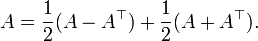is this the definition you are talking about?

where the first part is the skew-symmetric part and the second is the symmetric part?is this the definition you are talking about?

where the first part is the skew-symmetric part and the second is the symmetric part?

Actually, using AT = -A, or A + AT = 0

I have: (A+B)T = AT + BT = (-A) + (-B) = -(A+B)

So for the closure under multiplication, am I trying to show that kA is skew-symmetric or that (AB)T is skew-symmetric?

Thanks

Deveno
you need to show that kA is skew-symmetric if A is (we need closure under scalar multiplication, not matrix multiplication. matrix multiplication is not part of what makes nxn matrices a vector space, it's uh...erm...a bonus!).

you need to show that kA is skew-symmetric if A is (we need closure under scalar multiplication, not matrix multiplication. matrix multiplication is not part of what makes nxn matrices a vector space, it's uh...erm...a bonus!).

does anyone know how i can show that a skew-symmetric matrix contains the zero vector?

as for #3, i wrote my answer to include the zero vectors for u and v with w being my vector solution from finding the nullspace since u,v,w are linearly independent and span...

for #5 i was able to write (0,0,0) as a linear combination of the three vectors that were given (in terms of u, v and w).

Deveno
does anyone know how i can show that a skew-symmetric matrix contains the zero vector?

use the fact that 0T = 0....

as for #3, i wrote my answer to include the zero vectors for u and v with w being my vector solution from finding the nullspace since u,v,w are linearly independent and span...

never, never, never put a 0-vector in a basis. the 0-vector always makes ANY set linearly dependent.

for #5 i was able to write (0,0,0) as a linear combination of the three vectors that were given (in terms of u, v and w).

good :)

use the fact that 0T = 0....

never, never, never put a 0-vector in a basis. the 0-vector always makes ANY set linearly dependent.

good :)

awesome, thanks!!!!! :D